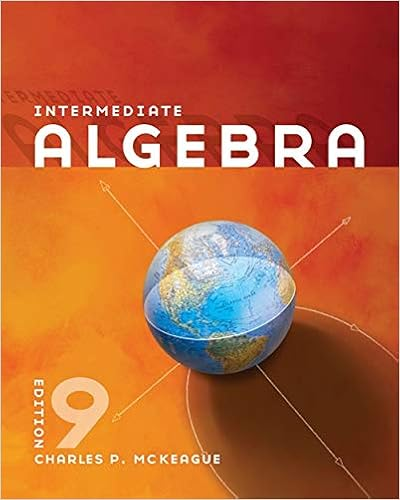# 128A_Final Practice Test - Alg Final Practice Test...

• Test Prep
• joshuagrandy
• 5

This preview shows page 1 - 3 out of 5 pages.

##### We have textbook solutions for you!
The document you are viewing contains questions related to this textbook.The document you are viewing contains questions related to this textbook.
Chapter 1 / Exercise 38
Intermediate Algebra
MckeagueExpert Verified
Alg: Final Practice Test Directions: Watch Practice Test Part 1 AND Part 2 Test reviews to answer all questions. ANSWER ALL PROBLEMS!SHOW ALL WORK! Print, show all work, scan and save to your computer as a PDF. Submit to the correct Assignment Basket.1.What is the conversion factor? Leave as a decimal. Do not round to the nearest whole number. a.) 332 inchesb.) 352 ounces c.) 5670 seconds2.Solve the linear equation 3. Solve the equation for y 3x+8y==z4. Graph the linear equation y35.Graph the linear equation 2x+6y=12=16 ounces = 1 pin
##### We have textbook solutions for you!
The document you are viewing contains questions related to this textbook.The document you are viewing contains questions related to this textbook.
Chapter 1 / Exercise 38
Intermediate Algebra
MckeagueExpert Verified
6. What graph represents the system oflinear inequalities7. Graph the function f(x)5x+y>1and y≤ x+3=
? 8.What is the graph of the piecewise-defined function f(x)?
•••